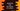# JavaScript program to find the volume of a sphere## JavaScript program to find the volume of a sphere:

In this post, we will learn how to find the volume of a sphere in JavaScript. With this program, you will learn how to do basic mathematical calculations in JavaScript.

To find the volume of a sphere, we need the radius. We can use just a simple formula to find the volume.

Below formula is used to find the volume of a sphere:

``Volume = 4/3 * πr^3``

Where, r is the radius of the sphere. π or PI is a mathematical constant. It is defined in the Math class in JavaScript. We can create one variable initialized with the value of π or we can simply use the Math class.

Now, to calculate the volume, we need just the radius of the sphere. We can then use this formula to find the volume.

In this post, we will learn different ways to solve this problem. Let’s check these one by one.

### Method 1: Finding the sphere volume with a given radius value:

Let’s try to find the volume of a sphere with a given radius value. The value of the radius is given and this program will simply print the volume of the sphere.

``````let radius = 5;
let volume = (4/3)* Math.PI * Math.pow(radius, 3);

console.log('Volume of Sphere: '+volume.toFixed(2));``````

Here,

• radius is the given radius of the sphere.
• The volume is holding the volume of the sphere. It uses the same formula we discussed above to calculate the volume.
• Finally, the last line is printing this value. It is formatting it to two digit after the decimal.

If you run this program, it will print the below output:

``Volume of Sphere: 523.60``

### Method 2: Finding the sphere volume with a user given radius value:

We can also take the radius as input from the user. The other part of the program will run in a same way. Just read the radius and calculate the volume.

``````let radius = parseInt(prompt("Enter the radius", "0"), 0);
let volume = (4/3)* Math.PI * Math.pow(radius, 3);

console.log('Volume of Sphere: '+volume.toFixed(2));``````

You need to run it in a browser. Go to the console tab in the developer menu and paste the code. It will ask the user to enter the radius value with a prompt. Enter it and hit ok, it will show the volume in the console.

You will get similar result as the above example.

### Method 3: Using with HTML:

We can also use the JavaScript code with a HTML page. Create one file called index.html with the below code:

``````<input type="number" id="input_radius" placeholder="Enter the radius">
<input type="button" value="Find" id="input_button" >
<script>

function calculateVolume(){

let volume = (4/3)* Math.PI * Math.pow(radius, 3);

alert("The volume of a sphere: "+volume.toFixed(2));
}

let button=document.getElementById("input_button");
button.onclick=calculateVolume;

</script>``````

This is a HTML file with the javascript code included to find the volume. If you open this file in a browser, it will show one input box with a button. This input box is to get the radius and the button is to calculate that value.

If you enter any value in that input box and click on the button, it will show one alert dialog that will show the volume of the sphere as like below:

You can add css to style the input box and the button as you like.